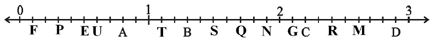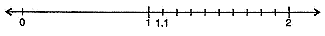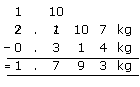# Extra Questions of Class 6 Decimals Chapter 8 Mathematics

Extra Questions of Class 6 Decimals Chapter 8 Mathematics. myCBSEguide has just released Chapter Wise Question Answers for class 6 Maths. There chapter wise Practice Questions with complete solutions are available for download in myCBSEguide website and mobile app. These Extra Questions with solution are prepared by our team of expert teachers who are teaching grade in CBSE schools for years. There are around 4-5 set of solved Mathematics Extra questions from each and every chapter. The students will not miss any concept in these Chapter wise question that are specially designed to tackle Exam. We have taken care of every single concept given in CBSE Class 6 Mathematics syllabus and questions are framed as per the latest marking scheme and blue print issued by CBSE for Class 6.

CBSE Class 6 Maths Extra Questions

## CBSE Class 6 Maths Chapter 8 Important Questions

Ch-8 Decimals

1. Write the decimal number represented by the points S on the given number line.1. 1.2
2. 1.6
3. 1.5
4. 1.4
2. 3 g = ________ kg
1. 0.03
2. 0.3
3. None of these
4. 0.003
3. Find: 0.29 + 0.36
1. 0.065
2. 0.65
3. None of these
4. 65
4. 31.24 + 7.03 = _______
1. zero
2. 38.07
3. 38.2
4. 38.27
5. 1.24 + 7.23 = _______
1. 8.45
2. 8.07
3. 8.4
4. 8.47
6. Match the following-

Column IColumn II
a. 2m 5cmi. 20 Rupees 15 paise
b. 14.035 kmii. Rs. 0.50
c. Rs 20.15iii. 2.05 m
d. 50 paiseiv. 14km 35m
7. Fill in the blanks.

1. {tex}25.135 = 25 + \frac{1}{{\__{}}} + \frac{3}{{\__{}}} + \frac{5}{{\__{}}}{/tex}
2. {tex}66.606 = 60 + \__{} + \frac{6}{{\__{}}} + 0 + \frac{6}{{\__{}}}{/tex}
3. {tex}14 + \frac{5}{{1000}} + \frac{6}{{10}} = 14.\__{}0\__{}{/tex}
4. {tex}\frac{4}{{100}} + 5 = \_\_.0\_\_{/tex}
8. State True or False:-

1. Rs. 60.75 can also expressed as 60Rs 75p.
2. Adding 70.25 and 14.6 gives 84.85.
3. 1 kg is equals to 100 grams.
4. Expanded form of 23.05 is {tex}20 + 3 + \frac{0}{{10}} + \frac{5}{{100}}{/tex}.
9. Arrange the following decimal numbers in descending order.
8.51, 8.06, 9.10, 8.57

10. Express {tex}\frac{6}{{100}}{/tex} as decimal.

11. Express the following as cm using decimals : 30 mm

12. Show 1.1 on the number line.

13. Express the following as cm using decimals
116 mm.

14. Subtract 0.314 kg from 2.107 kg.

15. Subtract the sum of 114.753 and 70.14 from the sum of 93.12 and 212.15.

Ch-8 Decimals

1. 1.5
Explanation: S is lies after 1 and fifth part toward right. So the decimal number represented by the points S on the given number line is 1.5.
1. 0.003
Explanation: 1000 g = 1 kg3 g = {tex}{3 \over {1000}}{/tex} kg= 0.003 kg
1. 0.65
Explanation: 0.29 + 0.36 = 0.65
1. 38.27
Explanation: 31.24 + 7.03 = 38.27
1. 8.47
Explanation: 1.24 + 7.23 = 8.47
1. {tex} \to {/tex} iii
100 cm = 1 m
so, 5 cm = {tex}\frac{5}{100}{/tex} m
Therefore, 2 m 5 cm = 2 m + {tex}\frac{5}{100}{/tex} m
= 2 m + 0.05 m
= 2.05 m
2. {tex} \to {/tex} iv
1 km = 1000 m
14.035 km = 14.035 {tex}\times{/tex} 1000 m
= 14035 m
= 14000 m + 35 m
= 14 km + 35 m = 14 km 35 m.
3. {tex} \to {/tex} i
1 Rupee = 100 paise
Rs. 20.15 = 20.15 {tex}\times{/tex} 100 p
= 2015 paise
= 2000 paise + 15 paise
= 20 Rupees + 15 paise = 20 Rupees 15 paise.
4. {tex} \to {/tex} ii
100 paise = 1 Rupee
50 paise = Rs. {tex}\frac{50}{100}{/tex} = Rs. 0.50.
1. 10, 100, 1000.
In the decimal number, 25.135, 1 is in tenths place. So its place value is {tex}\frac{1}{10}{/tex}, 3 is in hundredths place. So, its place value is {tex}\frac{3}{100}{/tex}.
6 is in thousandths place. So, its place value is {tex}\frac{6}{1000}{/tex}.
Therefore, {tex}25.135 = 25 + \frac{1}{10} + \frac{3}{100} + \frac{5}{1000}{/tex}
2. 6, 10, 1000
In the decimal number, 66..606, the place values of each 6 from left to right are: 60, 6, {tex}\frac{6}{10}{/tex}, {tex}\frac{0}{100}, \frac{6}{1000}{/tex}
Therefore, 66.606 = 60 + 6 + {tex}\frac{6}{10}{/tex} + 0 + {tex}\frac{6}{1000}{/tex}
3. 6, 5
The given expanded form 14 + {tex}\frac{5}{1000} + \frac{6}{10}{/tex} can be written in the correct order as, 14 + {tex}\frac{6}{10}+\frac{0}{100}+\frac{5}{1000}{/tex}
i.e., 6 is in tenths place, 0 is in hundredths place and 5 is in thousandths place.
So, the decimal number can be written as 14.605.
4. 5, 4
The given expanded form {tex}\frac{4}{100} + 5{/tex} can be written in the correct order as, 5 + {tex}\frac{0}{10} + \frac{4}{100}{/tex}.
i.e., 0 is in tenths place and 4 is in hundredths place. So, the decimal number can be written as 5.04.
1. True(Rs.60.75 = Rs 60 + Rs 0.75 = Rs 60 + Rs {tex}\frac{75}{100}{/tex}= 60Rs 75p)
2. True ( By changing both numbers in to like decimals and adding, 70.25 + 14.60 = 84.85)
3. False (We know that 1Kg = 1000g)
4. True ( The expanded form of 23.05 = 2 Tens + 3 Ones + 0 Tenths + 5 Hundredths = 20 + 3 + {tex}\frac{0}{10}{/tex} + {tex}\frac{5}{100}{/tex})
1. The given decimal numbers are like decimals. By checking the digits before decimal, we observe that 9.10 is largest of given numbers. Among the remaining numbers, by checking the tenths place, we observe that 8.06 is the least. Out of remaining numbers, by checking the hundredths place, we observe that 8.57 is bigger than 8.51. So, by arranging the given numbers from big to small, we have,
{tex} \Rightarrow {/tex} 9.10, 8.57, 8.51, 8.06
2. {tex}\frac{6}{100}{/tex} = 0.06
3. {tex}\begin{array}{l}\;1\;mm\;=\;\frac1{10}\;cm\\so\;30\;mm\;=\;\frac{30}{10}\;\;cm\\\;\;\;\;\;\;\;\;\;\;\;\;\;\;\;\;\;\;\;=\;\;3\;cm\;=\;3.0\;cm\;\end{array}{/tex}
4. We know that 1.1 is more than one but less than two. There is one-tenth in it. Divide the unit length between 1 and 2 into 10 equal parts and take 1 part as shown below :5. 116 mm = {tex}\frac{116}{10}{/tex} cm [{tex}\because{/tex} 1 mm {tex}=\frac{1}{10}{/tex}cm] {tex}=\frac{110+6}{10}{/tex} cm {tex}=\left(\frac{110}{10}+\frac{6}{10}\right){/tex}cm {tex}=\left(11+\frac{6}{10}\right){/tex}cm
= 11.6 cm
6. 0.314 kg from 2.107 kg = 1.793 kg7. Sum of 114.753 and 70.14:- {tex}\frac{{ + \begin{array}{*{20}{c}} {114.753} \\ {70.140} \end{array}}}{{184.893}}{/tex} Sum of 212.15 and 93.12:- {tex}\frac{{ + \begin{array}{*{20}{c}} {212.15} \\ {93.12} \end{array}}}{{305.27}}{/tex}
Difference in their sums = {tex}\frac{{ – \begin{array}{*{20}{c}} {305.270} \\ {184.893} \end{array}}}{{120.377}}{/tex}

## Chapter Wise Extra Questions for Class 6 MathematicsTest Generator

Create question paper PDF and online tests with your own name & logo in minutes.myCBSEguide

Question Bank, Mock Tests, Exam Papers, NCERT Solutions, Sample Papers, Notes

### 3 thoughts on “Extra Questions of Class 6 Decimals Chapter 8 Mathematics”

1. Thanks ??

2. Thank you so much learned a lot ?

3. ccc